Next: The NLO quasilocal functional Up: Time-dependent density functional theory Previous: Continuity equation for the

### Continuity equation for densities in spin-isospin channels

We can now repeat derivations presented in Eqs. (21)-(25) by considering the spin-isospin local-gauge groups, and derive CEs in other spin-isospin channels. To this end, we first express the nuclear one-body density matrix (13)-(14) as a linear combination of nonlocal spin-isospin densities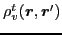[Carlsson and Dobaczewski(2010)],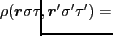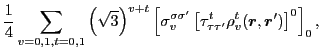(28)

where the sums run over the spin (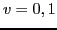) and isospin (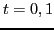) indices denoted by subscripts and superscripts, respectively, coupled to total scalar and isoscalar. Here and below we use the coupling of spherical tensors both for angular momentum and isospin tensors; therefore, in Eq. (28) the factor of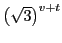was included so as to cancel the corresponding values of the Clebsch-Gordan coefficients, and to maintain the standard normalization of the spin-isospin densities. The spin-isospin densities can be conversely expressed as the following traces of the density matrix,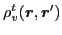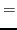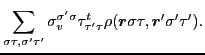(29)

The CEs for densities in the scalar-isoscalar (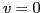,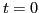), scalar-isovector (,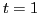), vector-isoscalar (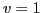,), and vector-isovector (,) channels,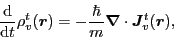(30)

where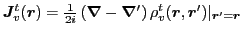and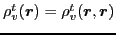, are now equivalent to the local gauge invariances, respectively, with respect to the four local spin-isospin groups: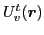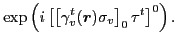(31)

Of course, the standard CE derived in Sec. 2.2.1 corresponds to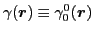. Note that the four gauge groups are different: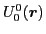gives the standard abelian gauge group U(1),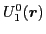and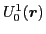form the non-abelian gauge groups SU(2), whereas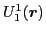corresponds to the non-abelian gauge group SU(2)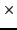SU(2).Next: The NLO quasilocal functional Up: Time-dependent density functional theory Previous: Continuity equation for the
Jacek Dobaczewski 2011-11-11